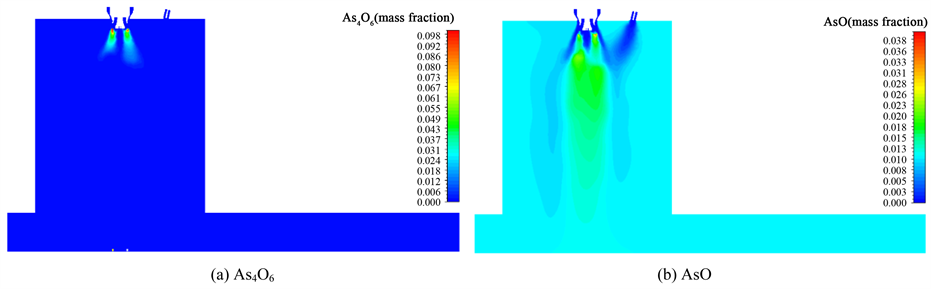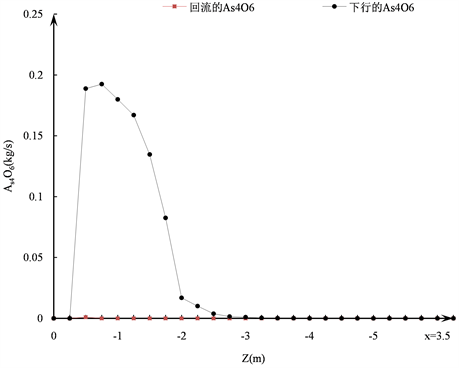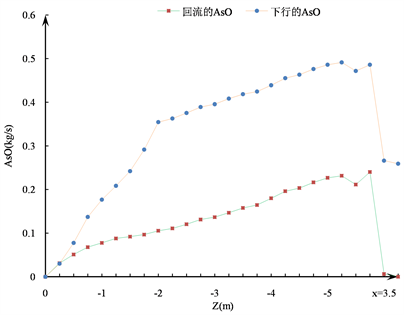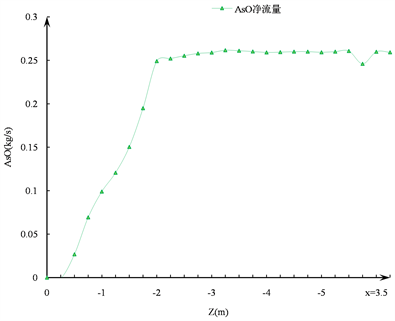# 铜闪速熔炼砷分配行为的数值仿真Numerical Simulation of Arsenic Distribution Behavior in Copper Flash Smelting

• 全文下载: PDF(1803KB)    PP.85-92   DOI: 10.12677/MEng.2018.52012
• 下载量: 552  浏览量: 1,481   科研立项经费支持

The numerical simulation model for the arsenic distribution behavior between gas and particle phases in a copper flash smelting process was established, and the distribution behavior was nu-merically simulated based on the software Fluent 6.3. The simulating results show that the arsenic was separated rapidly from Cu3AsS4 and As2O3 was quickly volatilized. The arsenic separation from the concentrate was almost completed within 3 m from the top of the reaction tower. The volatilization of As2O3 in the soot was finished within 1 m from the top of the reaction tower. The zone within 3 m from the top of the tower is the key to adjust the distribution of arsenic in each phase. For the simulated case, the proportion of arsenic distribution is 48.1% in the melt and 51.9% in the gas phase.

2. 物理数学模型

2.1. 物理模型

2.2. 数学模型Figure 1. Schematic diagram of copper flash furnace physical model. 1-Concentrate nozzle; 2-Gas burner; 3-React shaft; 4-Gas space of settler

${\text{2CuFeS}}_{\text{2}}+{\text{O}}_{\text{2}}={\text{Cu}}_{\text{2}}\text{S}+\text{2FeS}+{\text{SO}}_{\text{2}}$ (1)

${\text{FeS}}_{\text{2}}+{\text{O}}_{\text{2}}=\text{FeS}+{\text{SO}}_{\text{2}}$ (2)

$\text{3FeS}+{\text{5O}}_{\text{2}}={\text{Fe}}_{\text{3}}{\text{O}}_{\text{4}}+{\text{3SO}}_{\text{2}}$ (3)

${\text{2Cu}}_{\text{2}}\text{S}+{\text{3O}}_{\text{2}}={\text{2Cu}}_{\text{2}}\text{O}+{\text{2SO}}_{\text{2}}$ (4)

$\text{FeS}+{\text{3Fe}}_{\text{3}}{\text{O}}_{\text{4}}=10\text{FeO}+{\text{SO}}_{\text{2}}$ (5)

${\text{3Cu}}_{\text{2}}\text{O}+\text{FeS}=\text{6Cu}+\text{FeO}+{\text{SO}}_{\text{2}}$ (6)

${\text{4Cu}}_{\text{3}}{\text{AsS}}_{\text{4}}+{\text{13O}}_{\text{2}}={\text{6Cu}}_{\text{2}}\text{S}+{\text{As}}_{\text{4}}{\text{O}}_{\text{6}}+10{\text{SO}}_{\text{2}}$ (7)

${\text{2As}}_{\text{2}}{\text{O}}_{\text{3}}={\text{As}}_{\text{4}}{\text{O}}_{\text{6}}$ (8)

${\text{4Fe}}_{\text{3}}{\text{O}}_{\text{4}}+{\text{3As}}_{\text{4}}{\text{O}}_{\text{6}}+{\text{7O}}_{\text{2}}={\text{12FeAsO}}_{\text{4}}$ (9)

${\text{CH}}_{\text{4}}+{\text{2O}}_{\text{2}}={\text{CO}}_{\text{2}}+{\text{2H}}_{\text{2}}\text{O}$ (10)

${\text{As}}_{\text{4}}{\text{O}}_{\text{6}}=\text{4AsO}+{\text{O}}_{\text{2}},\text{\hspace{0.17em}}T>1073\text{\hspace{0.17em}}\text{K}$ (11)

2.3. 定解条件Table 1. Distribution parameters in flash furnaceTable 2. Batching parameters and characteristic particle size in flash furnaceTable 3. Phase composition of concentrates and sootTable 4. Phase composition of slag concentrate

3. 仿真结果与分析

3.1. 气相含砷物质的反应过程

As4O6和AsO在炉内的总体反应过程如图3所示。根据反应速率相对大小的不同，As4O6的生成与消耗过程可以分为以下三个阶段。

1) 生成速率大于消耗速率阶段：这一过程发生在距离塔顶0.75 m的区域内，此时精矿和配风刚入炉，温度较低，主要以As4O6的生成为主，在离塔顶0.75 m处时As4O6的量到达最大值。此时，虽然精矿还未着火，但在中央天然气燃烧产生的高温区域的作用下，部分As4O6开始分解，从图3(b)可以看出，在离塔顶0.75 m处时，炉内已经生成了一定的AsO。

2) 消耗速率大于生成速率阶段：这一过程发生在距离塔顶0.75~2.5 m的区域内，伴随着精矿的着火和中央天然气的燃烧，气相的温度急剧升高，在达到分解温度后，As4O6的生成速率便将大大低于其消耗的速率。图3(a)表明在距离塔顶2.5 m时，炉内原来积累的As4O6已消耗殆尽。

3) 生成速率等于消耗速率阶段：这一过程发生在距离塔顶2.5~3.25 m的区域，此时由于精矿已经完全着火并开始燃烧，气相的温度远超过As4O6的分解温度，致使As4O6在生成的一瞬间即被分解和消耗。Figure 2. Distribution of gas concentration field on the symmetry plane of flash smelting(a)(b)(c)

Figure 3. The trend of As4O6 and AsO in the furnace: (a) Generation and consumption trends of As4O6 in the furnace; (b) Trend of generation of AsO in the furnace; (c) Net flow of AsO in the furnace

3.2. 颗粒相含砷物质的反应过程Figure 4. Reaction of Cu3AsS4 and As2O3 (a) Cu3AsS4 mass distribution in mixed concentrates and soot; (b) Variation of Cu3AsS4 mass fraction along the height of the reactor; (c) As2O3 mass distribution in mixed concentrates and soot; (d) Variation of As2O3 mass fraction along the height of the reactor; (e) Mass distribution of FeAsO4 in mixed concentrates and fly ash;(f) Variation in the mass fraction of FeAsO4 along the height of the reactorTable 5. Distribution ratio of arsenic in each phase simulated

FeAsO4的生成反应如式(9)所示，生成过程如图4(e)、图4(f)所示。图4(e)表明，料柱中央的精矿颗粒FeAsO4的含量较高，而料柱外围的精矿颗粒FeAsO4的含量较低，这是因为位于塔中央的精矿颗粒更为集中，升温着火慢，粒径较小容易氧化造成的。从图4(f)可以看出：进入熔体的FeAsO4同进入气相的AsO一样，主要在距离塔顶3 m以上的区域内生成，而在3 m以下的区域，几乎不再有FeAsO4生成；精矿颗粒中FeAsO4含量的提高主要是由于精矿的进一步脱硫造成。

3.3. 砷在熔体与气相中的分布

4. 结论

1) Cu3AsS4的脱砷过程和As2O3的挥发过程都非常迅速，精矿中含砷物质Cu3AsS4的脱砷过程在距离反应塔顶3 m的区域内已基本完成，而烟灰中的含砷物质As2O3的挥发过程在距离反应塔顶1 m的区域内已基本完成；

2) 距离塔顶3 m以内的区域是调整砷进入熔体或气相比例的关键区域，闪速熔炼炉出口处砷在熔体中的分配比例为48.1%，在气相中的分配比例为51.9%。

NOTES

*通讯作者。

  易克俊. 砷在铜冶炼过程的分布及其控制[J]. 湖南有色金属, 2001, 17(z1): 1-2.  湛淑华. 影响钒触媒活性的因素[J]. 内蒙古石油化工, 2012(5): 40-41.  姚素平. 诱导法脱砷技术在铜电解液净化系统中的应用[J]. 有色金属(冶炼部分), 1996(1): 11-16.  Chaubal, P.C., Sohn, H.Y., George, D.B., et al. (1989) Mathematical Modeling of Minor-Element Behavior in Flash Smelting of Copper Concentrates and Flash Converting of Copper Mattes. Metal-lurgical and Materials Transactions B, 20, 39-51. https://doi.org/10.1007/BF02670348  Chen, C. and Ja-hanshahi, S. (2010) Thermodynamics of Arsenic in FeOx-CaO-SiO2, Slags. Metallurgical and Materials Transactions B, 41, 1165-1174. https://doi.org/10.1007/s11663-010-9430-0  Seo, K.W. and Sohn, H.Y. (1991) Mathematical Modeling of Sulfide Flash Smelting Process: Part III. Volatilization of Minor Elements. Metallurgical and Materials Transactions B, 22, 791-799. https://doi.org/10.1007/BF02651156  Chen, C., Zhang, L. and Jahanshahi, S. (2010) Thermodynamic Modeling of Arsenic in Copper Smelting Processes. Metallurgical and Materials Transactions B, 41, 1175-1185. https://doi.org/10.1007/s11663-010-9431-z  王云霄. 铜闪速炉分散风旋流喷吹方案的数值仿真与优化研究[D]: [硕士学位论文]. 长沙: 中南大学, 2011.  毛永宁. 高强度铜闪速熔炼过程数值仿真与操作参数配套方案优化研究[D]: [硕士学位论文]. 长沙: 中南大学, 2012.  戴扬. 基于数值仿真的铜闪速熔炼气粒传递过程强化技术研究[D]: [硕士学位论文]. 长沙: 中南大学, 2016.  周俊. 高强度闪速熔炼中的冶金过程研究[D]: [硕士学位论文]. 长沙: 中南大学, 2015.